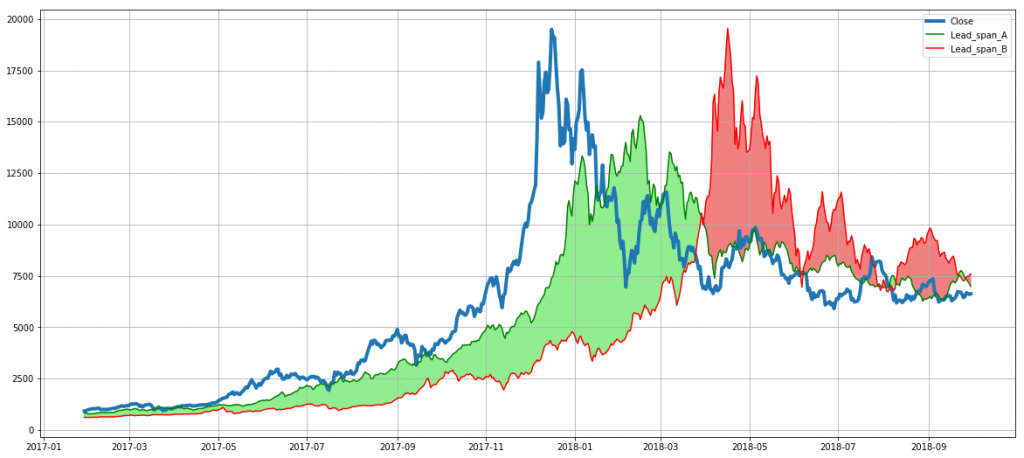# The Ichimoku Cloud And Trading StrategyBy Ashish Garg

The Ichimoku cloud indicator is a technical indicator of Japanese origin and was a proprietary indicator with its Japanese formulator for around 30 years.

It involves calculating five lines of short to medium duration on the high, low and close of a security’s prices and plotting an area, between two of these five lines, better known as Ichimoku cloud.

These lines help in determining the direction, momentum and support-resistance levels for the time series data. The Ichimoku cloud indicator also generates buy and sell trading signals and is usually plotted along with candlestick to enable better decision making and clearer plots.

Calculations for the lines are simple and the time period chosen is somewhat arbitrary:

1. Tenkan Sen or Conversion Line: (20-period-high + 20-period-low)/2
2. Kijun Sen or Base Line: (60-period-high + 60-period-low)/2
3. Senkou Sen A or Leading Span A: (Base Line + Conversion Line)/2
4. Senkou Sen B or Leading Span B: (120-period-high + 120-period-low)/2
5. Chikou Span or Lagging Span: (Close of 30 periods ago)

### The Direction Of The Price Action

The Ichimoku cloud is formed between the Leading Span A and Leading Span B and helps in determining the strength and direction of the price action. For example, the direction or trend of the price action is up when the prices are above the Ichimoku cloud. Similarly, the direction of the price action is down when prices are below the Ichimoku cloud and the direction is flat when the prices are somewhere in the Ichimoku cloud.

### The Strength Of The Price Action

The Strength can be estimated using the difference between the Leading Span A and B lines. When the Leading Span A is increasing and above the other span line, the increase in the difference signifies strength in the uptrend. It also means that the Ichimoku cloud is getting thicker. Similarly, the growth of the Leading span B over the other span line signifies strength in the downtrend and the thickness of the Ichimoku cloud increases again though in the opposite direction.

### Python Code And Trading Strategy

The Python code below loads the OHLC data for a cryptocurrency named Bitcoin. It then calculates, plots the various components and the Ichimoku cloud using the pandas and matplotlib functionality.
```## import packages
import pandas as pd
import matplotlib.pyplot as plt# read the excel; set ‘date’ column as index; rename the columns;
#discard columns other than Date and OHLC
df=pd.read_excel('BTC.xlsx',index_col = 'Date',names = ['Open','High','Low','Close'], usecols = range(5))
df.sort_index(inplace=True) ## Sort in chronological order or as earlier dates first
df=df.loc['2016-10-01':'2018-9-30'] ##Select some data using pandas’ .loc indexing# set values for the time periods to be used later
# becomes easier this way to change the time period values later to customise the calculations
CL_period = 20 # length of Tenkan Sen or Conversion Line
BL_period = 60 # length of Kijun Sen or Base Line
Lead_span_B_period = 120 # length of Senkou Sen B or Leading Span B
Lag_span_period = 30 # length of Chikou Span or Lagging Span# add to the dataframe, different components of the Ichimoku
# use shift function to shift a time series forward by the given value
df['Conv_line'] = (df.High.shift(CL_period)+df.Low.shift(CL_period))/2
df['Base_line'] = (df.High.shift(BL_period)+df.Low.shift(BL_period))/2
df['Lagging_span'] = df.Close.shift(Lag_span_period)
df.dropna(inplace=True) # drop NA values from Dataframe# plot the data using matplotlib's functionality
fig,ax = plt.subplots(1,1,sharex=True,figsize = (20,9)) #share x axis and set a figure size
ax.plot(df.index, df.Close,linewidth=4) # plot Close with index on x-axis with a line thickness of 4
ax.plot(df.index, df.Lead_span_A) # plot Lead Span A with index on the shared x-axis
ax.plot(df.index, df.Lead_span_B) # plot Lead Span B with index on the sahred x-axis# use the fill_between call of ax object to specify where to fill the chosen color
# pay attention to the conditions specified in the fill_between call